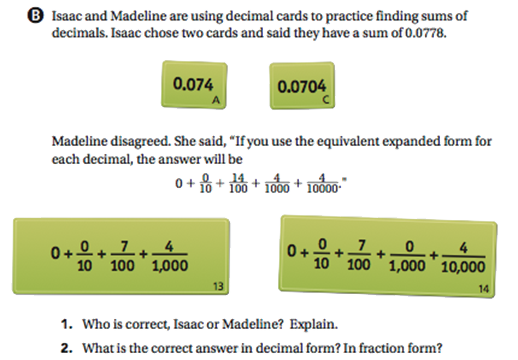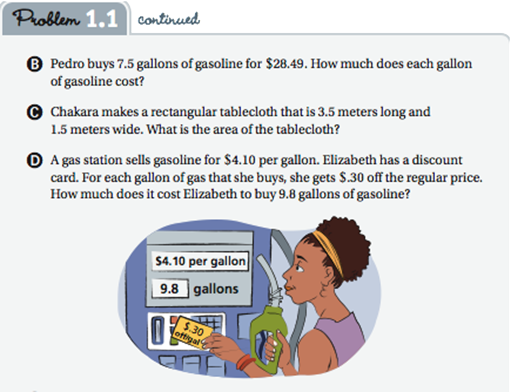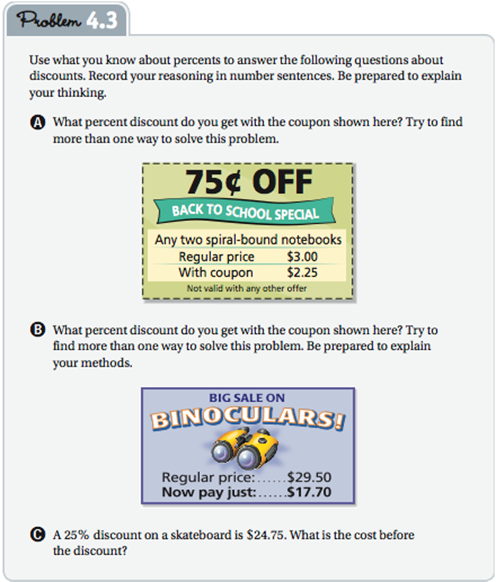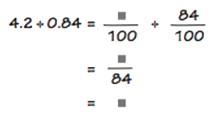Display Accessibility Tools

# CCSS.Math.Content.6.EE.A.1

Write and evaluate numerical expressions involving whole-number exponents.

## Decimal Ops Problem 2.1 implicit reasoning, explicit with extension

Evaluating numerical expressions involving exponents occurs explicitly in the Prime Time unit. The place value thinking throughout Decimal Ops incorporates the idea of exponents as powers of 10 as introduced in Comparing Bits and Pieces. For instance, in Problem 2.1 students use what they know about fractions to compare and add decimals. An extension that would make this powers of 10 use and reasoning explicit would be to rewrite some of the expanded forms in Problem 2.1 to include exponential notation. For example, a third question could be added here such as, “What is the correct answer in fraction form using powers of 10 in the denominator?” This type of thinking may help students later on when they study scientific notation.# CCSS.Math.Content.6.EE.A.2.a

Write expressions that record operations with numbers and with letters standing for numbers. For example, express the calculation "Subtract y from 5" as 5 - y.

## Decimal Ops Problems 1.1 and 4.3 plus extensions including variables.

Writing numeric and symbolic expressions and sentences to represent the operations required to solve problems is one of the most important skills in applied mathematics. Each unit of CMP3 includes many activities that develop understanding and proficiency in work on such modeling tasks. Problems 1.1 and 4.4 of Decimal Ops incorporate modeling tasks.

For instance, Problem 1.1 in Decimal Ops involves writing expressions to record problem-solving operations resulting in number sentences like:

9.8(4.10 – 0.30) = 37.24 [See problem 1.1 D]Using letter named variables to write expressions and equations representing quantitative operations and relationships is a primary focus throughout the Variables and Patterns unit. To get some head start on this important algebraic skill, the earlier exercises in writing numeric expressions and equations could be extended to suggest variables.

For instance, a question that asks, “Pedro buys 7.5 gallons of What is the cost per gallon of gasoline for \$28.49. How much does each gallon of gasoline cost?” [Decimal Ops 1.1 B above] could be extended to ask, “What formula tells the cost per gallon if 7.5 gallons cost d dollars?”

A question that asks, “What is the original price of an item if a 25% discount reduces that price by \$24.75 [Decimal Ops 4.3 C below] could be extended to ask, “What is the original price of an item if a 25% discount equals d dollars?”Understandings and skills used in writing numeric expressions and equations are also extended to use of letter names for variables in several other problems of Decimal Ops.

# CCSS.Math.Content.6.EE.A.2.b

Identify parts of an expression using mathematical terms (sum, term, product, factor, quotient, coefficient); view one or more parts of an expression as a single entity. For example, describe the expression 2 (8 + 7) as a product of two factors; view (8 + 7) as both a single entity and a sum of two terms.

## Decimal Ops Investigation 3

he mathematical terms sum, product, and factor are introduced early and used throughout Decimal Ops. The term quotient is defined and used in development of the decimal division algorithm in Decimal Ops Investigation 3, p. 48, and is used consistently throughout.

Each number in a division problem has a name to help you explain your thinking. The number you are dividing by is the divisor. The number into which you are dividing is the dividend. The answer is the quotient.

The calculation 18 divided by 3 equals 6 can be written like this:

18 ÷3 = 6

dividend ÷ divisor = quotient

You can also write the above calculation in two other ways:# CCSS.Math.Content.6.EE.A.2.c

Evaluate expressions at specific values of their variables. Include expressions that arise from formulas used in real-world problems. Perform arithmetic operations, including those involving whole-number exponents, in the conventional order when there are no parentheses to specify a particular order (Order of Operations). For example, use the formulas V = s3 and A = 6 s2 to find the volume and surface area of a cube with sides of length s = 1/2.

## Decimal Ops Problem 4.1 and 4.3 extensions

The obvious place where students develop skill in evaluating expressions for specific values of their variables is the measurement unit Covering and Surrounding. However, there are also opportunities in other CMP3 units. For example, Investigation 4 of Decimal Ops is all about applications of percents in which students are asked a variety of questions about sales tax and discounts on purchases. In answering those questions they are implicitly writing general formulas for calculating such taxes and discounts and then using the formulas in a number of specific cases. To more explicitly satisfy CCSS 6.EE.2a and 2c, you could ask students to write general formulas using letter names for variables and then ask them to show how to use those formulas in specific cases. Refer to the examples below for ideas on how to extend these problems.

## Problem 4.1 A extension

Write a number sentence for each question. Then answer the question.

A) Jill wants to buy an album of music that is priced at \$7.50. The sales tax is 6%. What will be the total cost of the album?

Try to find more than one way to solve this problem. Explain the different methods you find.

“What formula shows how to find the total price p of an item costing c dollars when
a tax of 6% must be added to the original cost.” [Ans: p = c + 0.06c or p = 1.06c]

## Problem 4.3 C (see p. 3 above) extension

“What formula shows how to calculate a 25% discount d on an item with original costc dollars?” [Ans: d = 0.25c]

# CCSS.Math.Content.6.EE.A.3

Apply the properties of operations to generate equivalent expressions. For example, apply the distributive property to the expression 3 (2 + x) to produce the equivalent expression 6 + 3x; apply the distributive property to the expression 24x + 18y to produce the equivalent expression 6 (4x + 3y); apply properties of operations to y + y + y to produce the equivalent expression 3y.

# CCSS.Math.Content.6.EE.A.4

Identify when two expressions are equivalent (i.e., when the two expressions name the same number regardless of which value is substituted into them). For example, the expressions y + y + y and 3y are equivalent because they name the same number regardless of which number y stands for.

## Decimal Ops Problem 4.1 and 4.3 extensions

In a similar manner to standard CCSS.Math.Content.6.EE.A.2.c above, some numeric calculation problems can be extended to generalize equivalent expressions. In this case, students learn that the total price can be represented by the equivalent expressions of c + 0.06c = 1.06c.

### Problem 4.1 A extension

#### Problem 4.1

Write a number sentence for each question. Then answer the question.

A) Jill wants to buy an album of music that is priced at \$7.50. The sales tax is 6%. What will be the total cost of the album?

Try to find more than one way to solve this problem. Explain the different methods you find.

“What formula shows how to find the total price p of an item costing c dollars when a tax of 6% must be added to the original cost.” [Ans: p = c + 0.06c or p = 1.06c]

# CCSS.Math.Content.6.EE.B.5

Understand solving an equation or inequality as a process of answering a question: which values from a specified set, if any, make the equation or inequality true? Use substitution to determine whether a given number in a specified set makes an equation or inequality true.

## Decimal Ops Problems 2.3 and 3.3

Students are engaged in solving equations and inequalities many times in 6th grade CMP3 units. For example, exercises in Investigations 2 and 3 of Decimal Ops ask students to “Find the value of n that makes n – 11.6 = 3.75 true” and “Find the value of N that makes N ÷ 08 = 3.5 true.” To emphasize these algebraic ideas, assign the associated ACE problems as well.

## Problem 2.3 and ACE #17, 18 and 19

### Problem 2.3

D) Use fact-family reasoning to find values of n that make these mathematical sentences true. Then check your answers.

1. n + 2.3 = 6.55
2. n + 6.88 = 7.21
3. 2n + 1.55 = 6.4

### ACE Problems

• 17. Find the value of n that make each sentence true. Then write the addition and subtraction fact family for the sentence.
1. 22.3 + 31.65 = n
2. 18.7 - 4.24 = n
• 18. Find the value of n that makes each sentence true. Use fact families to help.
1. 2.3 + n = 3.24
2. n - 11.6 = 3.75
• 19. Find the missing numbers.
1. 36.03 + n = 45.218
2. n + 0.488 = 13.762

## Problem 3.3 and ACE #30

### Problem 3.3

To find a quotient of decimals, you can think of the decimals as fraction.

• A) 1. ow might you complete the work below to find the quotient? Explain.• 2. Abe says that he uses multiplication to check his answers for division problems. He says, "4.2 ÷ 0.84" = n means that 0.84 goes into 4.2, n times. So, 0.84 × n = 4.2." Do you agree with Abe's reasoning? Does your answer for Question A, part (1), make Abe's multiplication sentence true? Explain.

### ACE Problem

• 30. Find the value of N that makes each equation true.
1. 3.2 × N = 0.96
2. 0.7 × N = 0.042
3. N × 3.21 = 9.63
4. N ÷ 0.8 = 3.5
5. 2.75 ÷ N = 5.5
6. 5.3N + 7.25 = 70.85

Explicit CMP3 attention to the meaning of solving an inequality does not occur until Variables and Patterns.

# CCSS.Math.Content.6.EE.B.6

Use variables to represent numbers and write expressions when solving a real-world or mathematical problem; understand that a variable can represent an unknown number, or, depending on the purpose at hand, any number in a specified set.

## Decimal Ops Problems 1.1 and 4.3 and 4.4 extensions

As indicated in the comments on Standard 2a, writing expressions and equations to represent conditions of real or purely mathematical questions occurs throughout the units of the CMP3 grade six course. Use of letter variables in such expressions occurs explicitly in the formulas of Covering and Surrounding and then throughout Variables and Patterns.

But there are also opportunities in other earlier units to extend numeric calculations and expressions by asking students for general expressions involving letter names for variables. For example, as noted in the remarks about Standards 6.EE.A.2a, 6.EE.A.3, and 6.EE.A.4, one can ask students to give general formulas for calculating sales tax or discounts when working with percents in Decimal Ops.

# CCSS.Math.Content.6.EE.B.7

Solve real-world and mathematical problems by writing and solving equations of the form x + p = q and px = q for cases in which p, q and x are all nonnegative rational numbers.

## Decimal Ops Problems 2.3 and 3.3 (See 6.EE.B.5 above)

Several CMP3 units develop student understanding and skill in work with these equations. The key idea in those earlier encounters with equation solving is fact families.

This use of fact families to solve one-step linear equations is developed and practiced in the Decimal Ops units. So students should have efficient and easy to recall strategies for solving x + p = q and px = q before they get to Variables and Patterns. Those early encounters with equation solving are generally embedded in the context of real world quantitative questions.

Note that the fact family way of thinking about and solving one-step linear equations is essentially an un-doing strategy. If one finds the 25% discount on an item by multiplying the original cost by 0.25, then to retrieve the original cost when the discount is known one divides the discount by 0.25.

The common equation solving strategy that relies on the idea of maintaining the equality of both sides of an equation (add, subtract, multiply, or divide equals by equals) is developed and applied later in Variables and Patterns.

# CCSS.Math.Content.6.EE.C.9

Use variables to represent two quantities in a real-world problem that change in relationship to one another; write an equation to express one quantity, thought of as the dependent variable, in terms of the other quantity, thought of as the independent variable. Analyze the relationship between the dependent and independent variables using graphs and tables, and relate these to the equation. For example, in a problem involving motion at constant speed, list and graph ordered pairs of distances and times, and write the equation d = 65t to represent the relationship between distance and time.

## Decimal Ops Problems 1.3 Introduces Unit Rate

This standard is essentially asking students to learn how to think about variables as quantities and equations as representations of functions or relationships.

In problem 1.3 of Decimal Ops, students explore the concept of unit rate, in each example setting up a natural opportunity to study relationships between a dependent variable and an independent variable linked by an equation in the form d = rt, with the coefficient r as the unit rate. In those situations thinking about unit rates is a natural invitation to thinking about how one variable changes as another related variable changes.

### 1.3 Take a Hike - Connecting Ratios, Rates and Decimals

The Madison Middle School Outdoor Club went on a three-day hiking trip in a national park. The club members made a table to show the distance traveled and time spent hiking for each of the three days.

Day Distance Traveled (miles) Time (hours)
1 9 4
2 12 5
3 6.4 2.5

How can you use the data above to compare the walking rates for each of the three days?

In Comparing Bits and Pieces, you learned about unit rates. You expressed each person's share of a chewy fruit worm as a unit rate. A unite rate is a comparison of two quantities in which one of the quantities being compared is 1 unit. In this Problem, you will use a calculator to find unit rates, such as the distance the hikers traveled per hour. You can express these unit rates in decimal form.